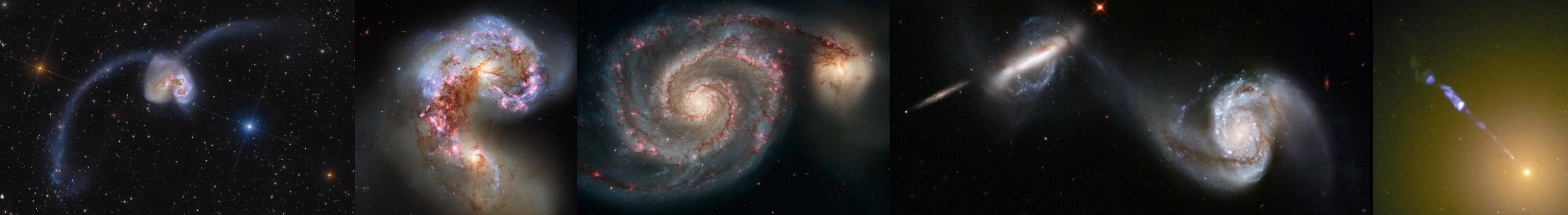# Paper: The interplay between AGN feedback and precipitation of the intracluster medium in simulations of galaxy groups and clusters

On this page you can find the supplementary material of for all three halo masses shown in the paper.

## The intermediate-mass halo at high time resolution

Here we show a movie for the intermediate-mass halo of 10^13.5 solar mass.

### The evolution of the halo on scales of 200 kpc

The different panels show the gas surface density (top left, within ± 25 kpc), mass-weighted temperature (top right, infinitely thin slice), mass-weighted pressure (bottom left, infinitely thin slice) and mass-weighted shock indicator (bottom right, infinitely thin slice)

## The most-massive halo

Here we show two movies for the most-massive halo of 10^14 solar mass halo.

### The evolution of the halo on scales of 200 kpc

The different panels show the gas surface density (top left, within ± 25 kpc), mass-weighted temperature (top right, infinitely thin slice), mass-weighted pressure (bottom left, infinitely thin slice) and mass-weighted shock indicator (bottom right, infinitely thin slice)

### The evolution of the radial profiles compared to the SFR

Here, I show the radial volume-weighted temperature (top left), volume-weighted hydrogen number density, volume-weighted entropy (middle left) and the ratio of the cooling time over the dynamical time (middle right). The bottom shows the star formation rate with the current time indicated by the blue line.

## The intermediate-mass halo

Here we show two movies for the intermediate-mass halo of 10^13.5 solar mass halo.

### The evolution of the halo on scales of 200 kpc

The different panels show the gas surface density (top left, within ± 25 kpc), mass-weighted temperature (top right, infinitely thin slice), mass-weighted pressure (bottom left, infinitely thin slice) and mass-weighted shock indicator (bottom right, infinitely thin slice)

### The evolution of the radial profiles compared to the SFR

Here, I show the radial volume-weighted temperature (top left), volume-weighted hydrogen number density, volume-weighted entropy (middle left) and the ratio of the cooling time over the dynamical time (middle right). The bottom shows the star formation rate with the current time indicated by the blue line.

## The low-mass halo

Here we show two movies for the low-mass halo of 10^13.5 solar mass halo.

### The evolution of the halo on scales of 200 kpc

The different panels show the gas surface density (top left, within ± 25 kpc), mass-weighted temperature (top right, infinitely thin slice), mass-weighted pressure (bottom left, infinitely thin slice) and mass-weighted shock indicator (bottom right, infinitely thin slice)

### The evolution of the radial profiles compared to the SFR

Here, I show the radial volume-weighted temperature (top left), volume-weighted hydrogen number density, volume-weighted entropy (middle left) and the ratio of the cooling time over the dynamical time (middle right). The bottom shows the star formation rate with the current time indicated by the blue line.